International
Tables for
Crystallography
Volume F
Crystallography of biological macromolecules
Edited by M. G. Rossmann and E. Arnold

International Tables for Crystallography (2006). Vol. F, ch. 19.4, p. 442   | 1 | 2 |

## Section 19.4.4. Distance measurements

D. M. Engelmana* and P. B. Mooreb

aDepartment of Molecular Biophysics and Biochemistry, Yale University, New Haven, CT 06520, USA, and  bDepartments of Chemistry and Molecular Biophysics and Biochemistry, Yale University, New Haven, CT 06520, USA
Correspondence e-mail:  don@paradigm.csb.yale.edu

### 19.4.4. Distance measurements

| top | pdf |

#### 19.4.4.1. Theory and background

| top | pdf |

By measuring distances between chemically specified points in a macromolecule or macromolecular complex, useful information may be obtained concerning its structure. In the case of a complex with many subunits, successive inter-subunit distance measurements can be combined by triangulation to generate a three-dimensional map specifying the relative positions of subunits and, perhaps, their individual radii of gyration. The only uncertainty is the handedness if more than three distances are combined. A number of approaches exist for making distance measurements, including NMR, fluorescence and electron-microscope techniques in which labelling is employed. Neutron scattering provides a particularly simple approach to such measurements in a conceptual sense. If we consider two labelled centres in a large macromolecule or complex, the interference of the scattering from the two labelled positions,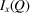, can be separated from the scattering of the rest of the complex by measuring the scattering of the unlabelled complex, I(Q), the two singly labelled complexes,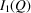and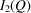, and the doubly labelled complex,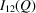, as they are related by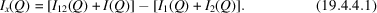Using the Debye relationship, it can be shown that the cross term will have the approximate form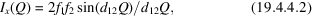where the interference intensity results from the correlation between radiation scattered from sites 1 and 2, with scattering strengthsand. It is a damped sinusoidal fringe with a periodicity reciprocally related to the separation between the scattering regions. If the scattering regions are very small compared with the object, there will be nodes at equal intervals in Q; if they are not,will be a sum of all cross correlations of label positions in the two regions.

#### 19.4.4.2. Neutron distance measurements

| top | pdf |

In the neutron case, the usual application involves deuterium labelling of positions in the two regions, and the cross term becomes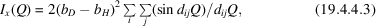where the first sum runs over all labelled positions in region 1 and the second over all labelled positions in region 2.

The interference experiment as a technique for studying macromolecules in solution was first proposed in 1947 as an X-ray scattering measurement in which the labels are heavy atoms (Kratky & Worthmann, 1947). It has been discussed several times since and tested (Hoppe, 1972; Vainshtein et al., 1970); however, no biological application has ever been published, since the signals are small (Hoppe, 1972). In 1972, the interference idea was discovered again, but in the context of neutron scattering (Engelman & Moore, 1972). Initially, it was thought that the distance measurement would follow from inspection of the damped sinusoidal term, but it soon became evident that the size and shape of the labelled regions would have an important influence. Using a power series expansion like that originally employed by Guinier, the previous expression becomes (for small Q)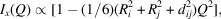whereandare the radii of gyration of the subunits in situ, andis the separation of their centres of mass. It follows that (Moore et al., 1978; Stöckel et al., 1979)The second moment of a length distribution,, can be related to a radius of gyration. If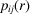is the length distribution of the cross term, then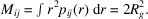Hence (Moore et al., 1978)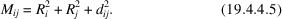Thus,is the parameter that contains the information in the difference experiment. If the radii of gyration of the labelled regions are small, then the distance is well measured in a single experiment. This would be the case, for example, if the labels were single heavy atoms in an X-ray experiment. However, in most complexes of macromolecules there will be many pairwise protein–protein relationships where the radii and the separation of centres have comparable magnitudes. One approach to extracting distance information is to know the radii of the subunits in situ, by estimation from their molecular weights, by measurement of the isolated subunits or from triple isotopic substitution (see above). The first approach is the least desirable but the easiest, and the last approach is correct but laborious.

If a complex has eight or more distinct subunits, the number of possible measurements of,, is sufficient to solve for the distances and radii, enriching the information obtained from the experiment. The minimum number of measurements required is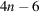, and for large complexes, such as the ribosome, a great excess of possible measurements exists, permitting refinement of the information through redundancy. Moore & Weinstein (1979) have described analytical methods for solving the problem with correct error propagation.

#### 19.4.4.3. The statistical labelling method

| top | pdf |

In many biological assemblies, multiple copies of a given subunit are found. In such cases, statistical labelling methods provide useful information. The basic concept is to produce two samples in which labelled subunits are randomly mixed with unlabelled subunits within the particles, and in which the ratios of labelled to unlabelled subunits is different. The difference in the scattering from the two samples will then contain shape and orientation information for the subunits (Fujiwara & Mendelson, 1996). A simple case is that of a dimer in a larger complex. Random introduction of equimolar labelled (D) and unlabelled (H) subunits will result in a mixture of complexes, where HH:HD:DH:DD = 1:1:1:1. A sample can be prepared with an equimolar mixture of all H and all D so that HH:DD = 1:1. The difference in scattering between these samples will be simply the interference cross term for the dimer, but at half the weight it would have in the case where the subunits were distinctly labelled.

### References

Engelman, D. M. & Moore, P. B. (1972). A new method for the determination of biological quarternary structure by neutron scattering. Proc. Natl Acad. Sci. USA, 69, 1997–1999.Google Scholar
Fujiwara, S. & Mendelson, R. A. (1996). In situ shape and distance measurements in neutron scattering and diffraction. Basic Life Sci. 64, 385–395.Google Scholar
Hoppe, W. (1972). New X-ray method for the determination of the quaternary structure of protein complexes. Isr. J. Chem. 10, 321–333.Google Scholar
Kratky, O. & Worthmann, W. (1947). Determination of the configuration of dissolved organic molecules by interferometric measurements with X-rays. Monatsch. Chem. 76, 263–281.Google Scholar
Moore, P. B., Langer, J. A. & Engelman, D. M. (1978). The measurement of the locations and radii of gyration of proteins in the 30S ribosomal unit of E. coli by neutron scattering. J. Appl. Cryst. 11, 479–482.Google Scholar
Moore, P. B. & Weinstein, E. (1979). On the estimation of the locations of subunits within macromolecular aggregates from neutron interference data. J. Appl. Cryst. 12, 321–326.Google Scholar
Stöckel, P., May, R., Strell, I., Cejka, Z., Hoppe, W., Heumann, H., Zillig, W., Crespi, H. L., Katz, J. J. & Ibel, K. (1979). Determination of intersubunit distances and subunit shape parameters in DNA-dependent RNA polymerase by neutron small-angle scattering. J. Appl. Cryst. 12, 176–185.Google Scholar
Vainshtein, B. K., Sosfenov, N. I. & Feigin, L. A. (1970). X-ray method for determining the gaps between heavy atoms in macromolecules in solution and its use for studying gramicidin derivatives. Dokl. Akad. Nauk SSSR, 190, 574–577.Google Scholar